# Mathematical EquationCartoons and Comics

## Mathematical Equation cartoon 1 of 11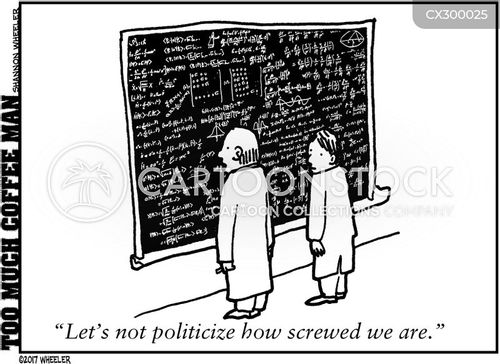"Let's not politicize how screwed we are."

Search ID:

CX300025

High Res:

4800x6600 (unwatermarked)

## Mathematical Equation cartoon 2 of 11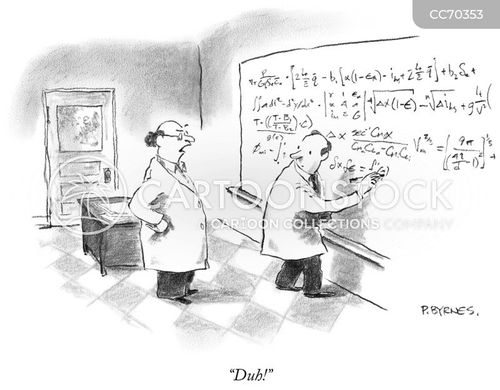"Duh!"

Search ID:

CC70353

High Res:

1561x2000 (unwatermarked)

## Mathematical Equation cartoon 3 of 11'The beauty of this is that it is only of theoretical importance, and there is no way it can be of any practical use whatsoever.'

Search ID:

shr1330

High Res:

2200x1828 (unwatermarked)

## Mathematical Equation cartoon 4 of 11My statistics are not rubbish. Why are you always so mean?

Artist:

Alborozo

Search ID:

galn16

High Res:

1813x1700 (unwatermarked)

## Mathematical Equation cartoon 5 of 11'That's it then - Time is money.'

Search ID:

ndw0274

High Res:

2383x2484 (unwatermarked)

## Mathematical Equation cartoon 6 of 11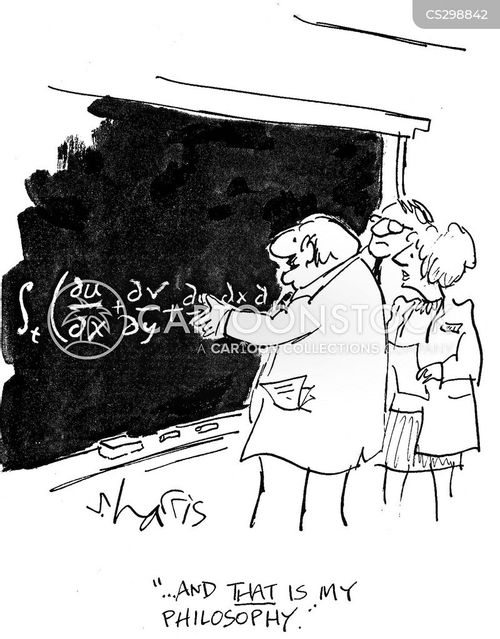'...and that is my philosophy.'

Search ID:

shrn346

High Res:

1876x1468 (unwatermarked)

## Mathematical Equation cartoon 7 of 11'I know! It's what I had last night for dinner.'

Search ID:

rmon954

High Res:

2956x2300 (unwatermarked)

## Mathematical Equation cartoon 8 of 11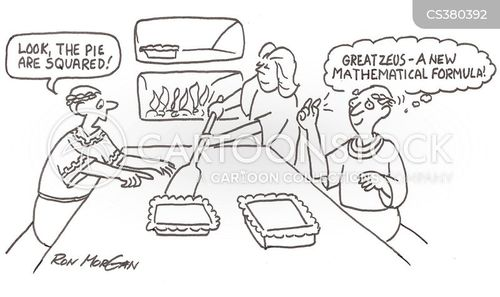Square pies produce new mathematical formula.

Search ID:

rmon953

High Res:

1776x2990 (unwatermarked)

## Mathematical Equation cartoon 9 of 11"You sure you don't want any Pi?"

Search ID:

cpa0071

High Res:

2099x2868 (unwatermarked)

## Mathematical Equation cartoon 10 of 11"Yo, this should be a two."

Search ID:

mgdn1558

High Res:

1500x2100 (unwatermarked)

## Mathematical Equation cartoon 11 of 11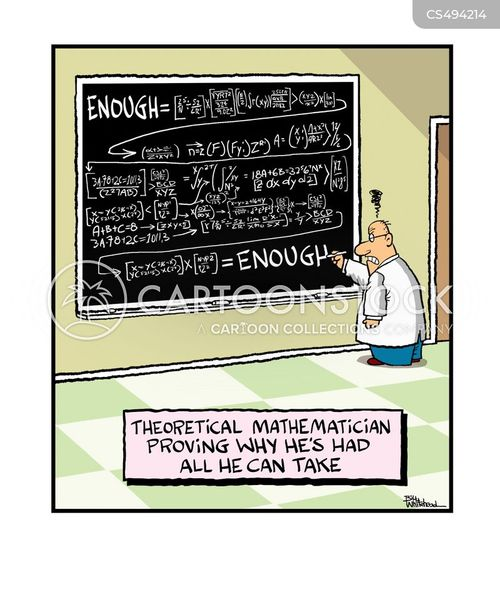Theoretical mathematician proving why he's had all he can take.

Search ID:

bwhn2537

High Res:

2700x2250 (unwatermarked)